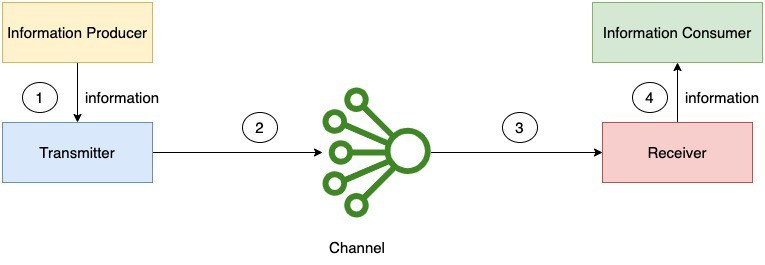## 1. Introduction

In this tutorial, we’ll briefly explain what information theory is about.

We can define it as a collection of mathematical and statistical laws about the transmission, storage, retrieval, and transformation of information. We use it in various scientific and engineering fields such as computer science, physics, quantum computing, communication engineering, molecular biology, social networks, and so on.

## 2. Brief History

We consider Claude Shannon as the father of classical information theory because he was the first person to quantity the information as produced by a source. In addition to him, we also give credit to Harry Nyquist. He is known for his sampling theorem, which forms the basis of communication system design.

Ralph Hartley also laid the foundation of communication science by discovering a relation between information rate, channel frequency, and transmission time. His discovery became one of the elements of Claude Shannon’s “A Mathematical Theory of Communication“. Additionally, he laid the foundations for modern-day radio direction finders that are used in ships and aircraft for navigation.

## 3. Information in Different Contexts

We can say that a piece of information refers to a set of processed data that was interpreted and given a definite meaning. However, its connotation changes in different fields and domains.

### 3.1. Information in Computer Science

From the perspective of computer science, a piece of information is organized and classified as data. It helps us draw crisp and concise inferences and guides us when taking business decisions. More so, information has 3Cs, namely complete, correct, and concise.

### 3.2. Information in Communication

In the field of communication, the information signals that we transmit between two entities over a communication medium. We say that communication is this process of transmission and reception of information:For example, when we make a mobile phone call, our voice is the information that we transmit over waves through the air as the medium or channel.

## 4. Information Theory

The basic idea behind information theory is that we can analyze information in the same way as any other physical quantity such as mass or energy. Thus, we can say that the information moves, transforms, and decays similarly to a fluid. Consequently, we can discover and formulate mathematical and physical laws characterizing each process involving information.

Information theory helps us define tight upper and lower bounds on the amount of information that can be communicated between any system’s two components. So, we can quantify any system’s efficiency using information theory.

Let’s consider a simple communication system with a transmitter sending information to a receiver over a communication channel. Any real-world channel will be far from perfect and will corrupt the information by adding various types of noise to it. Thus, channel noise reduces the efficiency of the channel as we can’t send complete information over it without corruption. We can use information theory rules to describe the channel precisely. That’s where entropy comes in.

### 4.1. Entropy

Entropy is the core concept of information theory. Entropy measures uncertainty.  Intuitively speaking, the more uncertain we are about something, the less information we have on it.

Formally, we can define entropyof a discrete random variablehavingstates as:

(1)whereis probability of. The units in which we express entropy are bits.

So, we find that a random variable representing only a single sure event (probability = 1) has the lowest entropy. On the other hand, a random variable whose all realizations are equally likely will have the largest entropy.

## 5. Applications of Information Theory

In information theory, we study optimal ways to store, compress, and transmit information. Thus, the field finds wide use from cryptography and genomics to linguistics, physiology, statistical inference, and beyond.

Let’s take a look at two examples.

### 5.1. Noisy Channel

Let’s say a communication channel is noisy and has the entropy ofbits with the capacity ofbits per second. As a result, we can use the channel for communication if and only if. Only in this case can we reliably encode, encrypt and send information over the channel using efficient coding techniques. Likewise, we can decrypt and decode it reliably at the receiver.

Why do we requireto be lower than? Well, what would happen otherwise? If, the noise present in the channel would corrupt the data since we couldn’t avoid channel errors regardless of the coding technique we use. In other words, the channel’s capacity limits the reliable information transmission rate.

### 5.2.  Machine Learning

Let’s consider the application of information theory in deep learning. Let’s say we want to train a Convolutional Neural Network (CNN) to classify input images. To do so, we can use the cross-entropy loss function.

The cross-entropy is a concept from information theory. Formally, we can define cross entropybetween two random variablesandhaving probability distributionsandon each outcomeas:

(2)It quantifies the difference betweenand.

## 6. Conclusion

In this article, we briefly explored the world of information theory and its application in various fields. Information theory is deeply mathematical and statistical. It is the pillar of modern-day communication and encryption systems and is also finding widespread use in machine learning.